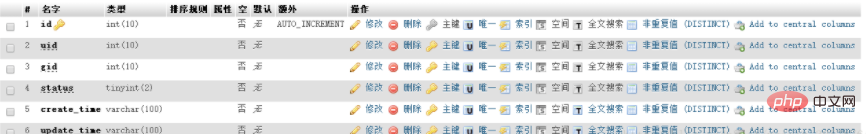php实现收藏功能```<script>
\$(function(){
\$('#sc').click(function(){
var gid=\$(this).attr('data-id');
var data={
gid:gid
};
\$.ajax({
type:"post",
data:data,
success:function(data){
// window.clearInterval(timer);
if(data==1){
}else {
if(data==2){
\$('#sc').css({
'background-color':'white',
'color':'#00ccff',
});
\$('#sc_words').html(
'收藏'
);
}else if(data==3){
\$('#sc').css({
'background-color':'#00ccff',
'color':'white',
});
\$('#sc_words').html(
'已收藏'
);
}else{
}
}
},
error:function(){
}
});
});
})
</script>```

html部分

```      <div id="sc" data-id="{\$detail.id}" class="in_right">
<p id="sc_words">收藏</p>
</div>```

php后台处理

```  // 商品收藏   1 代表未登入 2代表取消收藏  3 代表 收藏成功
if(empty(session('uid'))){
echo '1';
}else {
\$collect=M('collect');
\$gid=I('post.gid');
//先確定是否已收藏
\$map['gid']=\$gid;
\$map['uid']=session('uid');
\$data=\$collect->where(\$map)->find();
if(\$data){
if(\$data['status']==1){
\$collect->where('id='.\$data['id'])->setField('status',0);
echo '2';
}else{
\$collect->where('id='.\$data['id'])->setField('status',1);
echo '3';
}
}else{
if(\$collect->create()){
\$collect->gid=\$gid;
\$collect->create_time=get_date();
\$collect->uid=session('uid');
\$collect->status=1;